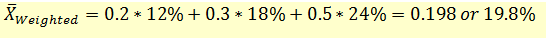# Calculating Weighted Average Mean

One characteristic of an arithmetic mean is that all observations have equal weight (=1/N). However, this may not always be the case. In some cases, different observations may influence the mean differently. This has special relevance in portfolios where a portfolio is made up of different stocks each having a different weight.

Let’s assume that we have a portfolio comprising three stocks, A, B and C as follows:

 Stock Returns Weight A 12% 20% B 18% 30% C 24% 50%

We have the stock returns for each stock and the weight of each stock in the portfolio. For example, if the investor has a total of $1,000 invested in the portfolio, 20% or$200 is invested in Stock A, $300 is invested in stock B, and the remaining$500 is invested in Stock C.

The weighted average mean is calculated using the following formula:The weighted mean of our portfolio will be calculated as follows:Note that the weighted mean is closer to the returns from Stock C because Stock C has more influence (weight) on the portfolio.

Get our Data Science for Finance Bundle for just $29$51.